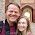## Friday, October 5, 2012

### <-s and =s and assigns in R

# I think it worth going into how R uses uses arrows and equal signs as well as the "assign" command.

# A single left arrow acts the same as a single equal sign.

# Thus:

x=4
x

y<-x
y

# This basically says take the value of x and assign it to y.  Interestingly the arrows can go either direction.

y->z
z

# They can also be stacked

a<-b<-c<-d<-y
# Is the same as:
a=b=c=d=y
# As well as:
y->a->b->c->d
a
b
c
d

# Sometimes it is useful and necessary to use a double arrow.  This means assign to the surface environment the variable value.

# This is important in function some times though most skilled programmers would probably recommend against too frequent use.

# Imagine if we run the function fx we want the variable ZZ to be assigned a value of 34.
fx <- function() {ZZ <- 34}

fx()
ZZ
# However, ZZ cannot be found.  This is because as default functions only create temporary variables that do not affect the variables available in the end user's environment.

# This can be gotten around by using double arrows.

fz <- function() {ZZ <<- 34}

fz()
ZZ

# Another useful way of assigning values to variable names is through the assign command.

# Assign takes a text and assigns a value to it as a variable.  Thus:

assign("aa", 434)
aa

# This can be very useful when you would like to generate many variables.

for(i in 1:10) assign(paste("a",i,sep=""),i^3)

# This will create 10 variables a1 a2 a3 etc with values equal to 1^2 2^2 3^3 etc.

a1
a2
a3
a10

# One tricky thing that I do not yet understand is how to assign values within an array.

# For instance:
a<-1:10
a

# Now I want a=100
assign("a",100)
a
# This is weird to me.

# The complementry function with the assign function is the get function.

get("a")

# But strangely:
get("a")

# Is different from:
get("a")

# Perhaps someone who knows R better than I can give me some advice in this matter.  This is a problem that I have frequently encountered when coding.

1.1.